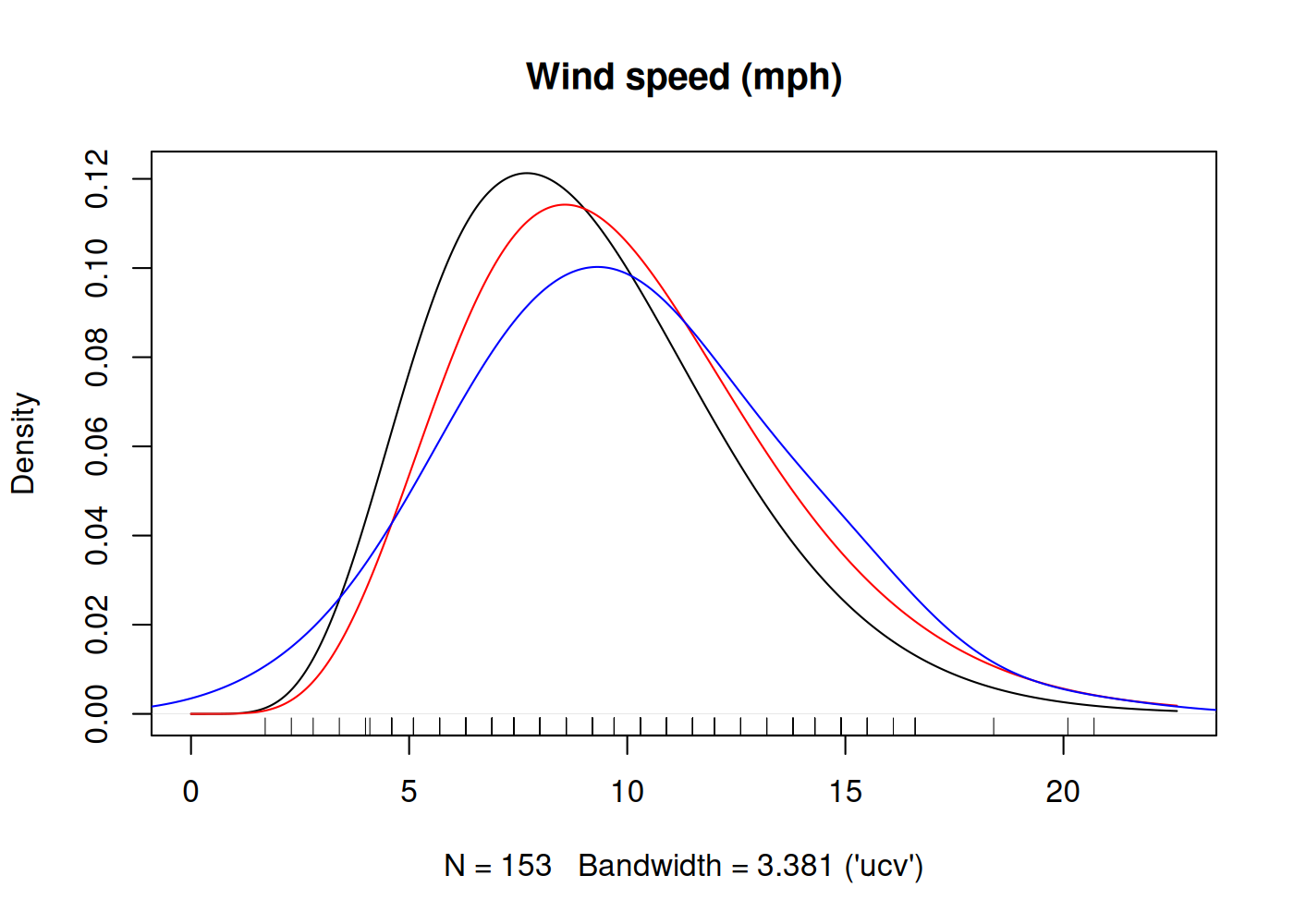Kernel Density Estimation with Parametric Starts and Asymmetric Kernels

Handles univariate non-parametric density estimation with parametric starts and asymmetric kernels in a simple and flexible way. Kernel density estimation with parametric starts involves fitting a parametric density to the data before making a correction with kernel density estimation, see Hjort & Glad (1995) . Asymmetric kernels make kernel density estimation more efficient on bounded intervals such as (0, 1) and the positive half-line. Supported asymmetric kernels are the gamma kernel of Chen (2000) , the beta kernel of Chen (1999) , and the copula kernel of Jones & Henderson (2007) . User-supplied kernels, parametric starts, and bandwidths are supported.

An R package for univariate kernel density estimation with parametric starts and asymmetric kernels.

Overview

kdensity is an implementation of univariate kernel density estimation with support for parametric starts and asymmetric kernels. Its main function is kdensity, which is has approximately the same syntax as stats::density. Its new functionality is:

• kdensity has built-in support for many parametric starts, such as normal and gamma, but you can also supply your own.
• It supports several asymmetric kernels ones such as gcopula and gamma kernels, but also the common symmetric ones. In addition, you can also supply your own kernels.
• A selection of choices for the bandwidth function bw, again including an option to specify your own.
• The returned value is callable: The density estimator returns a density function when called.

A reason to use kdensity is to avoid boundary bias when estimating densities on the unit interval or the positive half-line. Asymmetric kernels such as gamma and gcopula are designed for this purpose. The support for parametric starts allows you to easily use a method that is often superior to ordinary kernel density estimation.

Installation

First you need to install the package devtools from CRAN. From inside R, use the following command.

This installs the latest version of the package from GitHub. Call the library function and use it just like stats:density, but with optional additional arguments.

Description

Kernel density estimation with a parametric start was introduced by Hjort and Glad in Nonparametric Density Estimation with a Parametric Start (1995). The idea is to start out with a parametric density before you do your kernel density estimation, so that your actual kernel density estimation will be a correction to the original parametric estimate. This is a good idea because the resulting estimator will be better than an ordinary kernel density estimator whenever the true density is close to your suggestion; and the estimator can be superior to the ordinary kernal density estimator even when the suggestion is pretty far off.

In addition to parametric starts, the package implements some asymmetric kernels. These kernels are useful when modelling data with sharp boundaries, such as data supported on the positive half-line or the unit interval. Currently we support the following asymmetric kernels:

These features can be combined to make asymmetric kernel densities estimators with parametric starts, see the example below. The package contains only one function, kdensity, in addition to the generics plot, points, lines, summary, and print.

Usage

The function kdensity takes some data, a kernel kernel and a parametric start start. You can optionally specify the support parameter, which is used to find the normalizing constant.

The following example uses the data set plots both a gamma-kernel density estimate with a gamma start (black) and the the fully parametric gamma density. The underlying parameter estimates are always maximum likelood.Since the return value of kdensity is a function, it is callable, as in:

You can access the parameter estimates by using coef. You can also access the log likelihood (logLik), AIC and BIC of the parametric start distribution.

Reference manual

install.packages("kdensity")

1.1.0 by Jonas Moss, a year ago

https://github.com/JonasMoss/kdensity

Report a bug at https://github.com/JonasMoss/kdensity/issues

Browse source code at https://github.com/cran/kdensity

Authors: Jonas Moss , Martin Tveten

Documentation:   PDF Manual